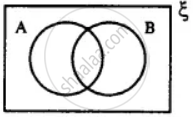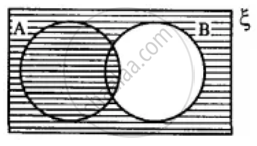# In the Given Diagram, Shade the Region Which Represents the Set Given Underneath the Diagrams: (B - A)' - Mathematics

Diagram

In the given diagram, shade the region which represents the set given underneath the diagrams: (B - A)'#### Solution

(B - A)' =Concept: Venn Diagram
Is there an error in this question or solution?

#### APPEARS IN

Selina Concise Mathematics Class 8 ICSE
Chapter 6 Sets
Exercise 6 (E) | Q 9.1 | Page 76